Next: Indirect calibration Up: Uncertainty due to systematic Previous: Correction for known systematic   Contents

## Measuring two quantities with the same instrument having an uncertainty of the scale offset

Let us take an example which is a little more complicated (at least from the mathematical point of view) but conceptually very simple and also very common in laboratory practice. We measure two physical quantities with the same instrument, assumed to have an uncertainty on the zero'', modelled with a normal distribution as in the previous sections. For each of the quantities we collect a sample of data under the same conditions, which means that the unknown offset error does not change from one set of measurements to the other. Calling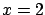and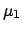the true values,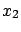and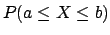the sample averages,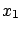and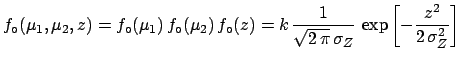the average's standard deviations, and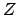the true value of the zero'', the initial probability density and the likelihood are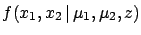(5.72)

and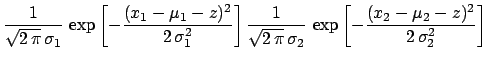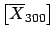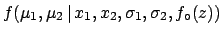(5.73)

respectively. The result of the inference is now the joint probability density function ofand: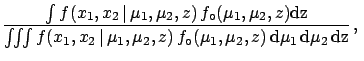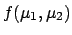(5.74)

where expansion of the functions has been omitted for the sake of clarity. Integrating we get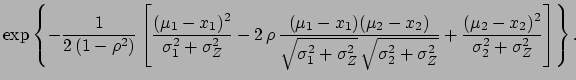(5.75)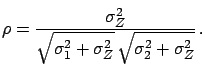where(5.76)

If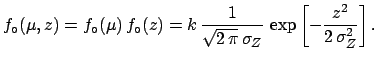vanishes then () has the simpler expression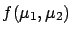(5.77)

i.e. if there is no uncertainty on the offset calibration then the joint density function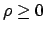is equal to the product of two independent normal functions, i.e.andare independent. In the general case we have to conclude the following.
• The effect of the common uncertaintymakes the two values correlated, since they are affected by a common unknown systematic error; the correlation coefficient is always non-negative (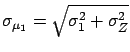), as intuitively expected from the definition of systematic error.
• The joint density function is a multinormal distribution of parameters,,,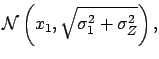, and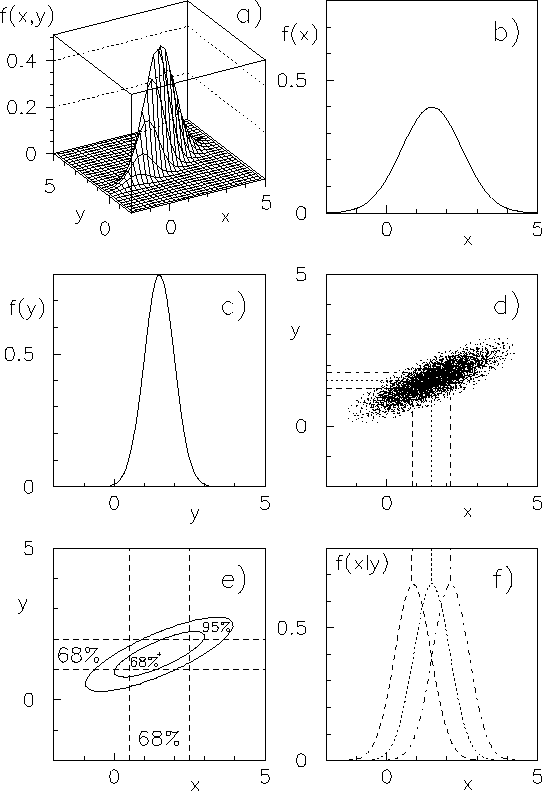(see example of Fig.).
• The marginal distributions are still normal: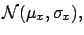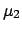(5.78)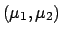(5.79)

• The covariance betweenandis
 Cov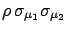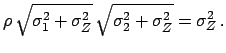(5.80)

• The distribution of any function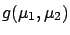can be calculated using the standard methods of probability theory. For example, one can demonstrate that the sumand the difference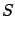are also normally distributed (see also the introductory discussion to the central limit theorem and sectionfor the calculation of averages and standard deviations):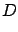(5.81)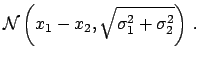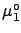(5.82)

The result can be interpreted in the following way.
• The uncertainty on the difference does not depend on the common offset uncertainty: whatever the value of the true zero'' is, it cancels in differences.
• In the sum, instead, the effect of the common uncertainty is somewhat amplified since it enters in phase'' in the global uncertainty of each of the quantities.Next: Indirect calibration Up: Uncertainty due to systematic Previous: Correction for known systematic   Contents
Giulio D'Agostini 2003-05-15